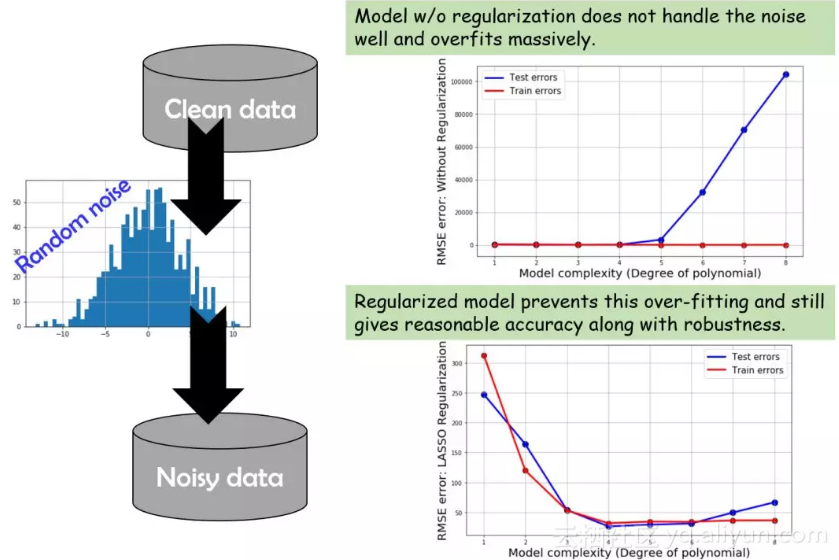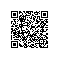# 用Python进行机器学习（附代码、学习资源）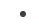怎么确定拟合多项式的顺序？是否需要为多变量回归加上交叉耦合项？有没有简单的方法将这一过程自动化？怎样判断模型是否过拟合？如何得知模型在面对噪声时够不够稳健？模型能不能轻松拓展到更高维度或更大的数据集上？

“我们能不能画出数据图形直接得到结论？”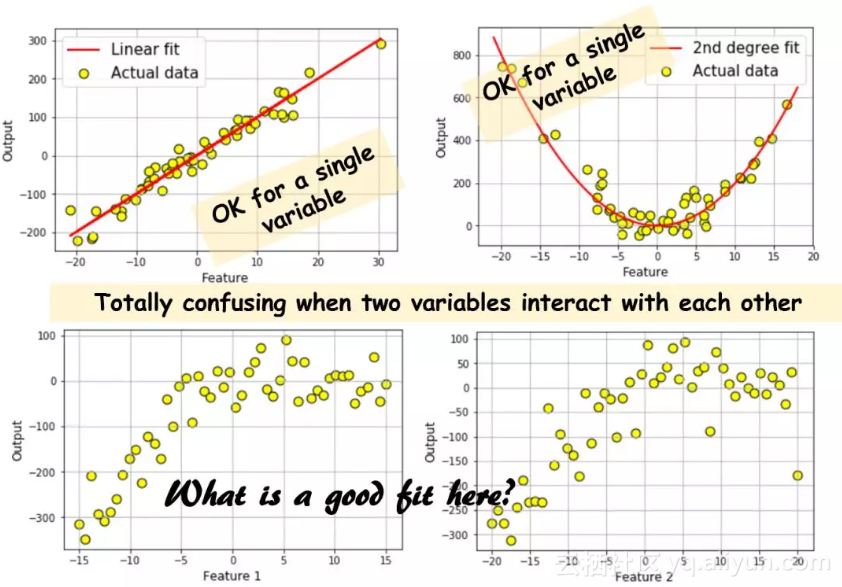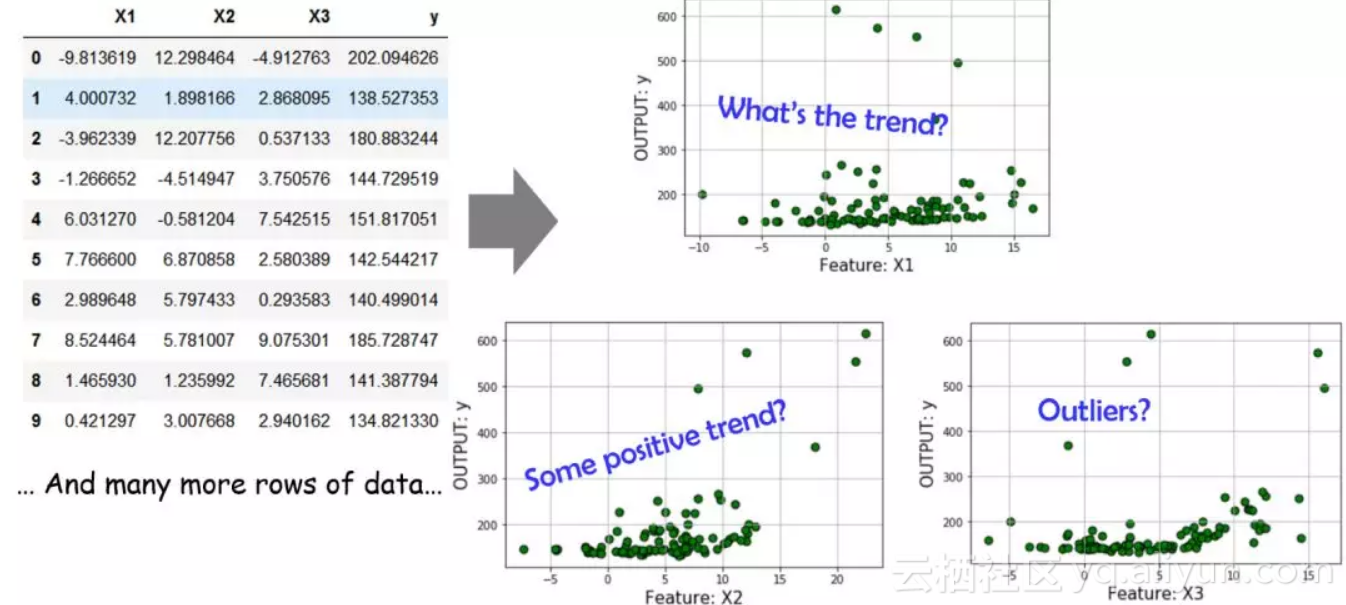怎样确定什么多项式是有用的？如果我们开始将一次项、二次项、三次项...逐个进行组合，什么时候停止比较合适呢？我们怎么判定哪些交叉耦合的项是重要的？比如：是只需要_X_1²、_X_2³还是需要有_X_1._X_2以及_X_1².X3这种项？最后，我们是不是必须手动将这些多项式转换的方程/函数式写出来并且应用到数据集上？

http://scikit-learn.org/stable/modules/generated/sklearn.linear_model.LinearRegression.html#sklearn.linear_model.LinearRegression

https://towardsdatascience.com/simple-and-multiple-linear-regression-in-python-c928425168f9?gi=69160943145f

from sklearn.cross_validation#引入函数进行训练集和测试集的划分

import train_test_split

#引入函数自动生成多项式特征

from sklearn.preprocessing import PolynomialFeatures

# 引入线性回归和一个正则化的回归函数

from sklearn.linear_model import LinearRegression

from sklearn.linear_model import LassoCV

from sklearn.pipeline import make_pipeline

Scikit-learn提供了一个从一组线性特征中生成多项式特征的方法。你需要做的就是传入线性特征列表，并指定希望生成的多项式项的最大阶数。它还可以让你选择是生成所有交叉耦合项还是只生成主要特征的阶数。这里有一个Python代码进行演示。

http://scikit-learn.org/stable/auto_examples/linear_model/plot_polynomial_interpolation.html#sphx-glr-auto-examples-linear-model-plot-polynomial-interpolation-py

https://www.analyticsvidhya.com/blog/2016/01/complete-tutorial-ridge-lasso-regression-python/对上述概念总结的一个Quora回答：

https://www.quora.com/What-is-a-pipeline-and-baseline-in-machine-learning-algorithms另外一篇关于机器学习流水线的总结：

https://medium.com/@yanhann10/a-brief-view-of-machine-learning-pipeline-in-python-5f50b941fca8对piplines在实践中重要性作出阐述：

https://www.oreilly.com/ideas/building-and-deploying-large-scale-machine-learning-pipelinesScikit-learn提供了一个流水线功能，可以将多个模型和数据预处理类组合在一起，把原始数据转换为可用模型。

http://scikit-learn.org/stable/tutorial

/statistical_inference/putting_together.html

# LASSO回归的参数设置

lasso_eps = 0.0001

lasso_nalpha=20

lasso_iter=5000

# 多项式特征项的最大、最小阶数

degree_min = 2

degree_max = 8

# 训练集、测试集划分

X_train, X_test, y_train, y_test =

train_test_split(df['X'], df['y'],test_size=test_set_fraction)

# 建立一个流水线模型

for degree in range(degree_min,degree_max+1):

model=make_pipeline(PolynomialFeatures(degree, interaction_only=False),LassoCV(eps=lasso_eps,

n_alphas=lasso_nalpha,max_iter=lasso_iter,

normalize=True,cv=5))

model.fit(X_train,y_train)

test_pred = np.array(model.predict(X_test))

RMSE=np.sqrt(np.sum(np.square(test_pred-y_test)))

test_score = model.score(X_test,y_test)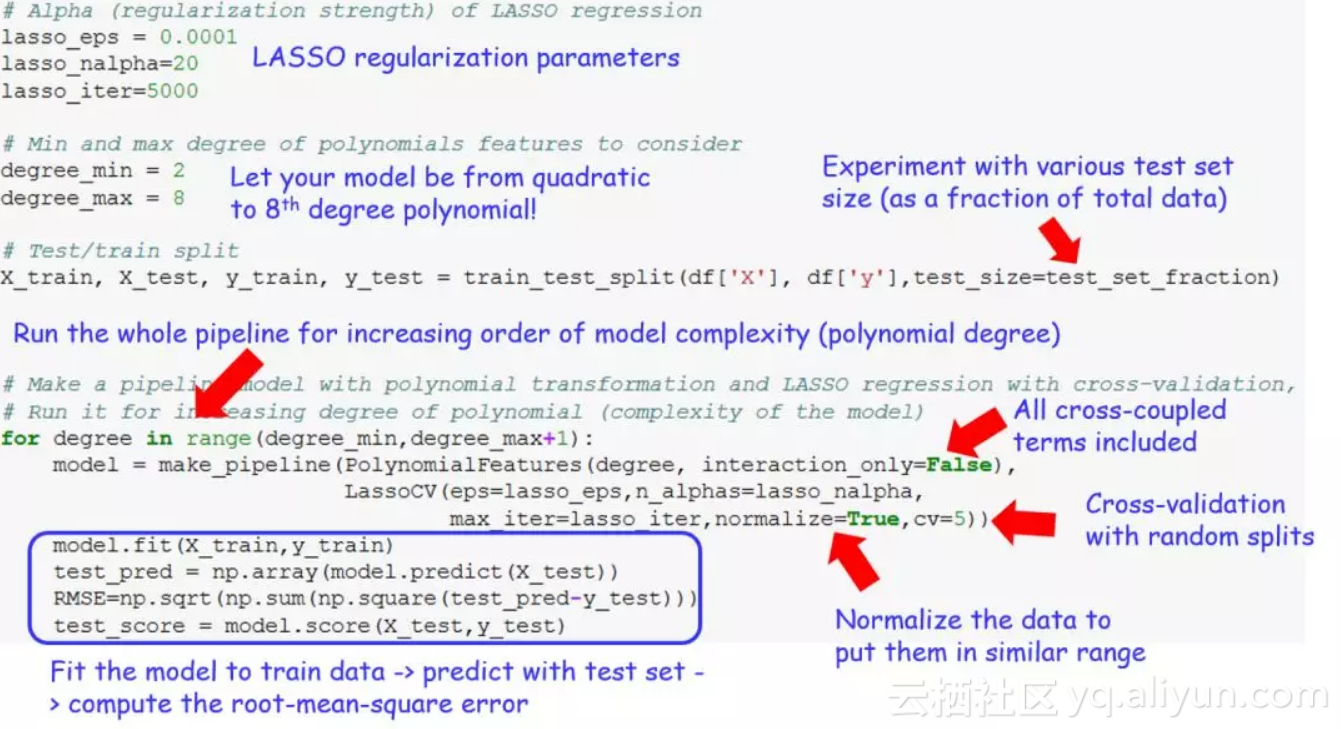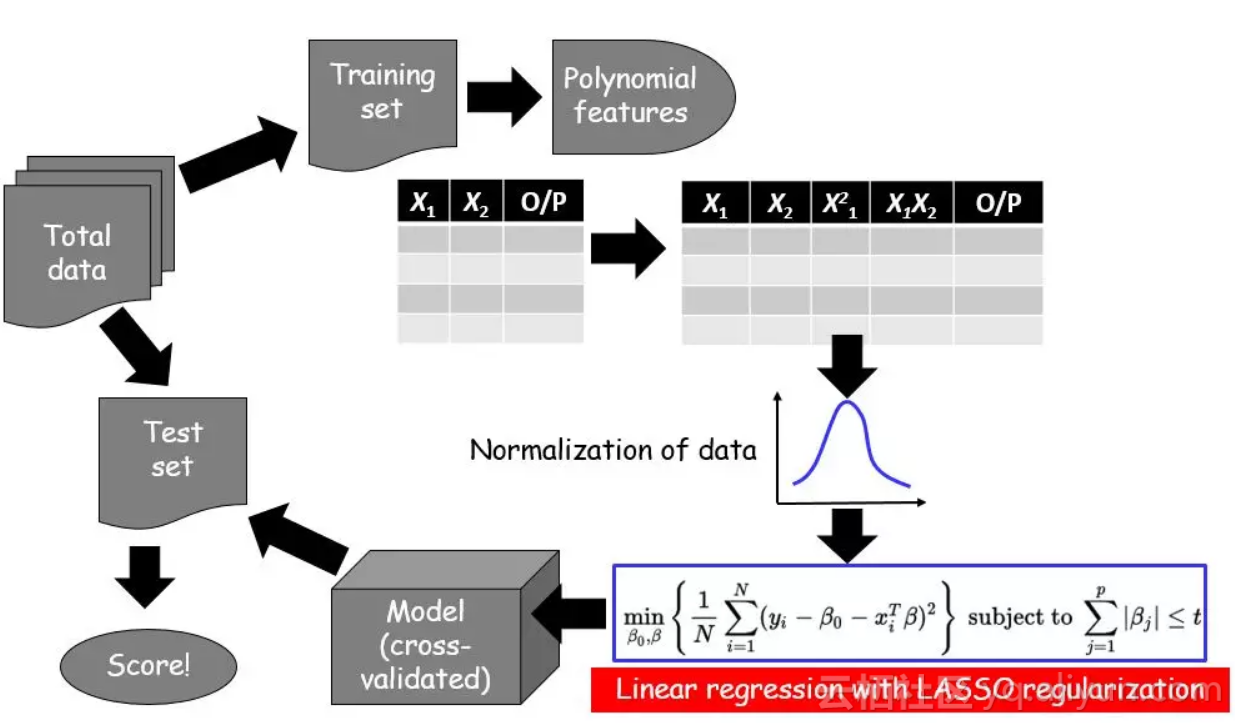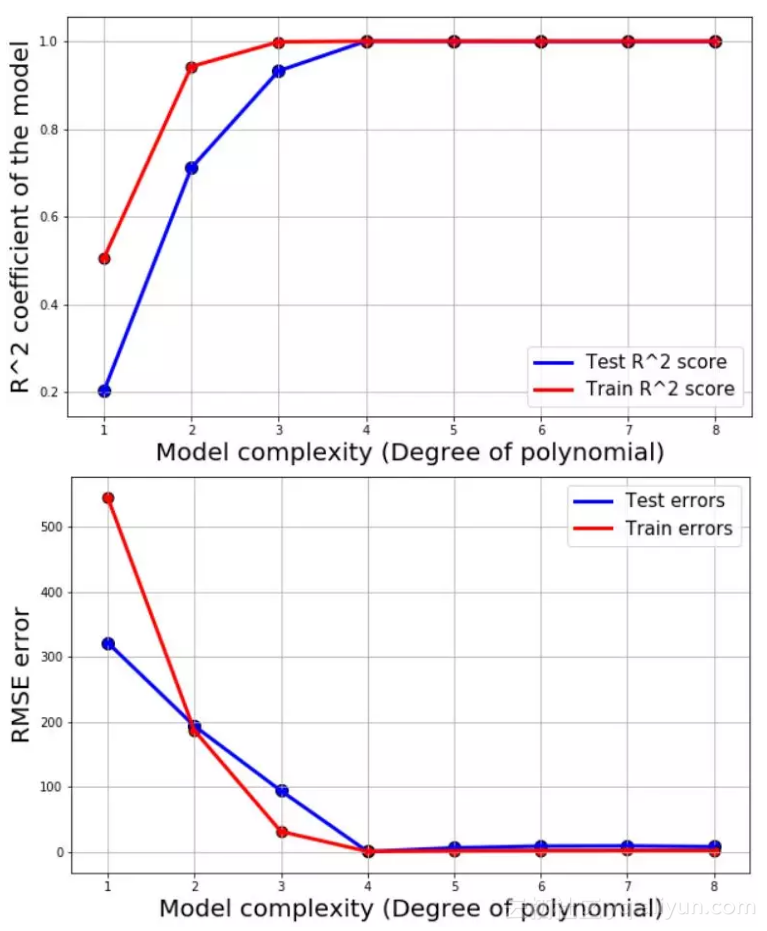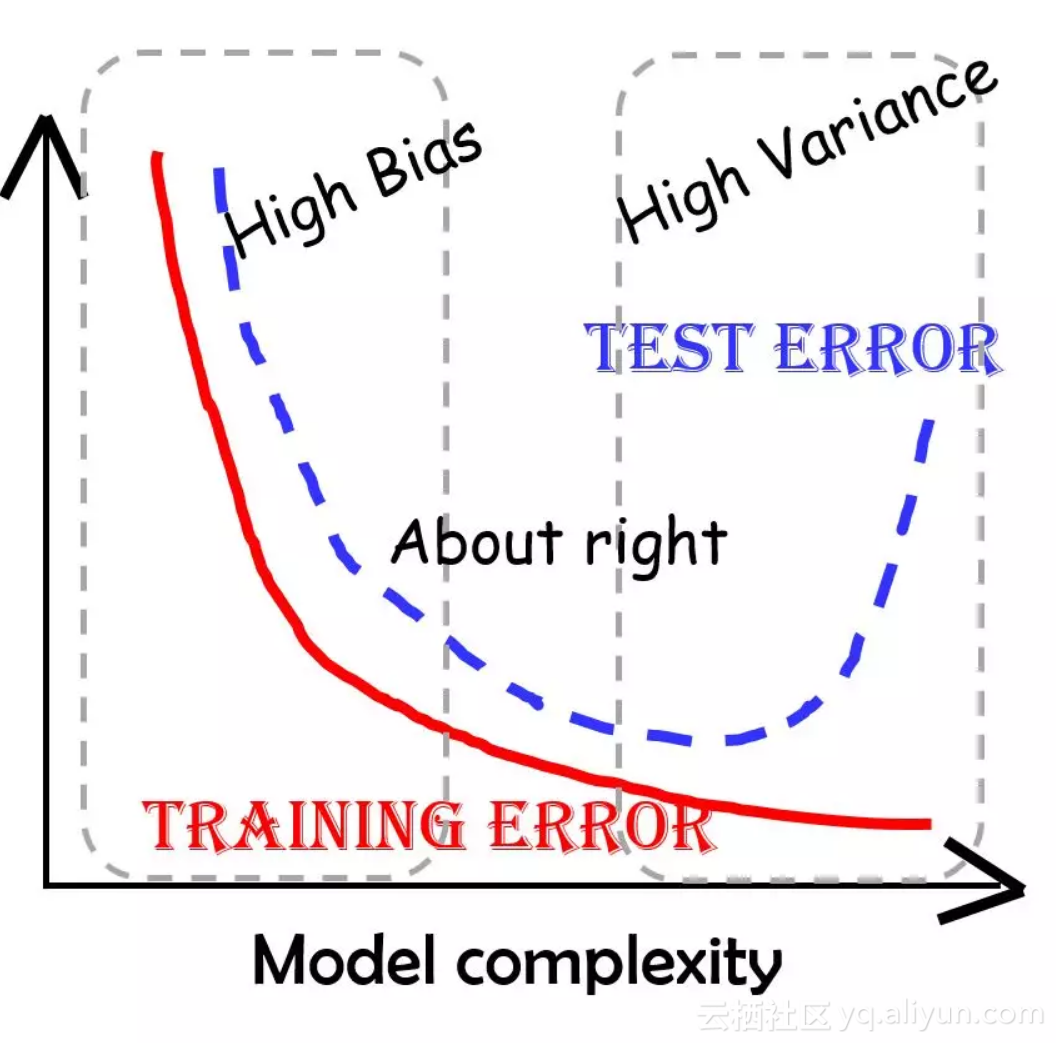https://dataorigami.net/blogs/napkin-folding/79033923-least-squares-regression-with-l1-penalty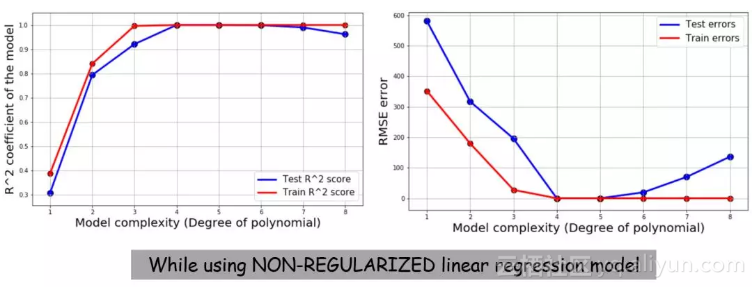（这里作者有提到自己的代码，同样因为GitHub地址失效没有加进来）